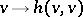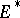# Negative vector bundle

(diff) ← Older revision | Latest revision (diff) | Newer revision → (diff)
A holomorphic vector bundle (cf. also Vector bundle, analytic)over a complex spacethat possesses a Hermitian metricsuch that the functiononis strictly pseudo-convex outside the zero section (this is denoted by). The vector bundleis negative if and only if the dual vector bundle(see Positive vector bundle). Ifis a manifold, then the condition of being negative can be expressed in terms of the curvature of the metric. Any subbundle of a negative vector bundle is negative. A vector bundleover a complex manifold is said to be negative in the sense of Nakano ifis positive in the sense of Nakano. A holomorphic vector bundleover a compact complex spaceis said to be weakly negative if its zero section possesses a strictly pseudo-convex neighbourhood in, i.e. ifis weakly positive. Every negative vector bundle overis weakly negative. Negative and weakly negative linear spaces over a spaceare also defined in this way.